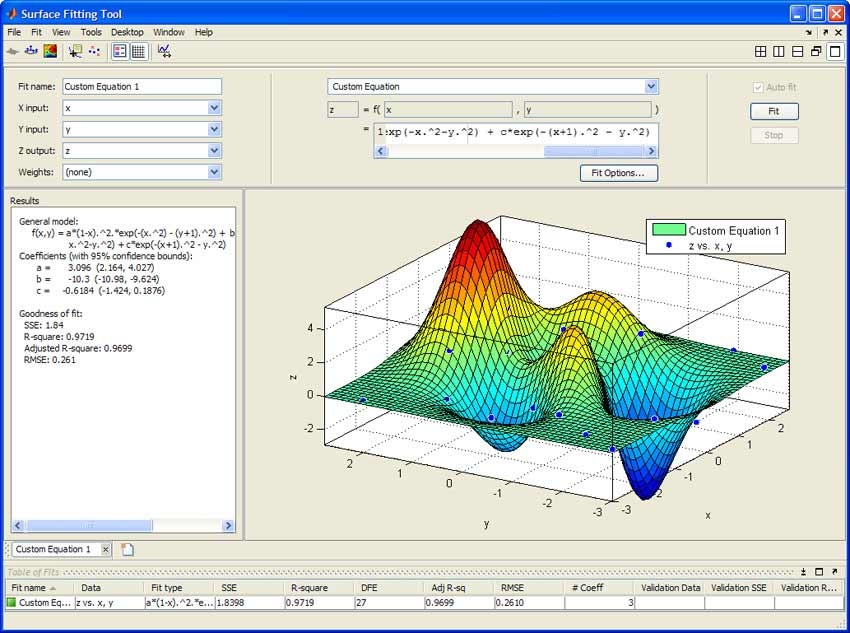## Plants resting on a table

In a previous post I wrote about fidelity in plant models.  For this post I want to focus, briefly, on three fundamental plant modeling tools that can be used to create simple and fast plant models.

1. Table-lookup functions and adaptive tables
2. Regression functions
3. Transfer functions

## Lookup tables

Lookup tables form the backboneof many plant models.  From thermodynamic property lookups to engine torque and power curves they allow users to quickly access multi-input / single output data.

The efficiency and memory usage of tables can be be dramatically improved when the conditions of their use is well understood.  Common methods include

1. Index search from last index:  In cases of slow moving data it is faster to start the indexing into the table by starting from the last time steps value.
2. Reuse of index across multiple tables:  In many instances the same X or Y axis will be used across multiple tables.  The calculation cost for finding the index can be decreased through a pre-lookup function.
3. Chose the correct interpolation function:  The efficiency of tables is dependent on the interpolation function.
4. Pick and condition your data:  Frequently data from real world measurements is not evenly spaced.  By preconditioning the data (e.g. smoothing out data and providing even spacing for axis…)

## Regression functions

Regression functions allow for modeling of non-linear multi-variable functions.  Two cautions regression functions.  First is in ensuring that data is valid throughout the input variable range and that the accuracy is sufficient at all points in the range.The second caution is to validate that the regression equation does not become computational burdensome.

## Transfer functions

The final “simple” plant model that I want to cover is the use of transfer functions.  Transfer functions can be used to model a system when the mathematical equations describing the system are know and involve state (e.g. derivative) information.  (Note for multi-input, multi-output functions State Space equations can be used)

Transfer functions have the advantage over table look ups and regression functions that the represent the actual underlying physical system from a theoretically correct perspective.   In some instances the function may need to be “tuned” to take into accounts imperfections in real world systems.

## Final thoughts

In the end there are many different methods for creating plant models.  Frequently the “correct” approach is to use a combination of “simple” and “advanced” modeling methods.  Take the time to think about what you require from your plant and the correct combinations can be selected.## 3 thoughts on “Plants resting on a table”

This site uses Akismet to reduce spam. Learn how your comment data is processed.